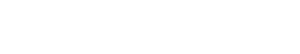# Binomial Test

Binomial Test is a method used to assess whether a given word or phrase is statistically significant. This test is often used to determine if a word or phrase is predictive of a certain outcome, and can be used to compare the performance of different models.

The purpose of Binomial Test for text analytics is to determine if a given word or phrase is predictive of a certain outcome. For example, if we are interested in predicting whether a customer will purchase a product, we might use the Binomial Test to assess whether the word “buy” is predictive of purchase. If the test shows that the word “buy” is statistically significant, then we would conclude that it is predictive of purchase. However, if the test does not show that the word “buy” is statistically significant, then we would conclude that it is not predictive of purchase.

The Binomial Test can be used to compare the performance of different models. For example, if we are interested in predicting whether a customer will purchase a product, we might use the Binomial Test to assess whether model A is more predictive of purchase than model B. If the test shows that model A is more predictive than model B, then we would conclude that it is a better predictor of purchase. However, if the test does not show that model A is more predictive than model B, then we would conclude that model A is not a better predictor of purchase.

The Binomial Test is a powerful tool for text analytics, but it is important to remember that it has some limitations. First, the Binomial Test can only be used to compare two groups. Second, the Binomial Test assumes that the data are Normally distributed. Violations of this assumption can lead to inaccurate results.

## When to use Binomial tests in text analytics

The Binomial Test is a powerful tool that can be used to assess the predictive power of a given word or phrase. This test is most useful when you have a dichotomous outcome (i.e., two groups).

The Binomial Test is also a good choice when you want to compare the performance of two models. For example, if you are interested in determining which of two models is better at predicting whether a customer will purchase a product, the Binomial Test can help you answer this question.

When deciding whether or not to use the Binomial Test, it is important to keep in mind that this test has some limitations. First, the Binomial Test can only be used to compare two groups. Second, the Binomial Test assumes that the data are Normally distributed. Violations of this assumption can lead to inaccurate results.

Despite its limitations, the Binomial Test is a valuable tool that can be used to assess the predictive power of a given word or phrase. When used correctly, the Binomial Test can help you answer important questions about your data.

## How to perform a Binomial test in text analytics

One way is to use a statistical software package, such as R or SAS.Unlock the power of actionable insights with AI-based natural language processing.